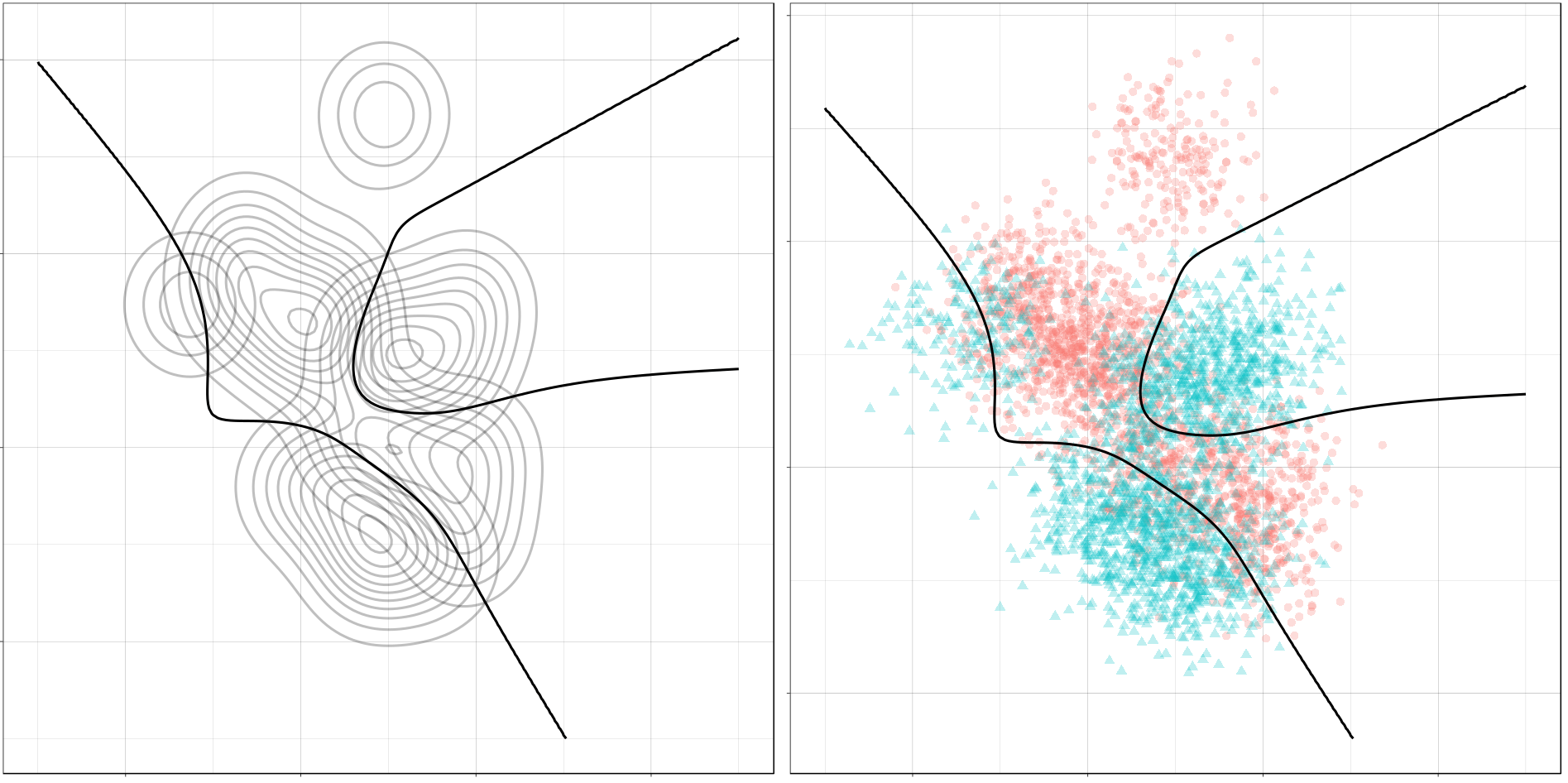Tag: modeling# Animated Machine Learning Classifiers

Ryan Holbrook made awesome animated GIFs in R of several classifiers learning a decision rule boundary between two classes. Basically, what you see is a machine learning model in action, learning how to distinguish data of two classes, say cats and dogs, using some X and Y variables.

These visuals can be great to understand these algorithms, the models, and their learning process a bit better.

Here’s the original tweet, with the logistic regression animation. If you follow it, you will find a whole thread of classifier GIFs. These I extracted, pasted, and explained below.

Below is the GIF which I extracted using EZgif.com.

What you see is observations from two classes, say cats and dogs, each represented using colored dots. The dots are placed along X and Y axes, which represent variables about the observations. Their tail lengths and their hairyness, for instance.

Now there’s an optimal way to seperate these classes, which is the dashed line. That line best seperates the cats from the dogs based on these two variables X and Y. As this is an optimal boundary given this data, it is stable, it does not change.

However, there’s also a solid black line, which does change. This line represents the learned boundary by the machine learning model, in this case using logistic regression. As the model is shown more data, it learns, and the boundary is updated. This learned boundary represents the best line with which the model has learned to seperate cats from dogs.

Anything above the boundary is predicted to be class 1, a dog. Everything below predicted to be class 2, a cat. As logistic regression results in a linear model, the seperation boundary is very much linear/straight.Logistic regression gif by Ryan Holbrook

These animations are great to get a sense of how the models come to their boundaries in the back-end.

For instance, other machine learning models are able to use non-linear boundaries to dinstinguish classes, such as this quadratic discriminant analysis (qda). This “learned” boundary is much closer to the optimal boundary:Quadratic discriminant analysis gif by Ryan Holbrook

Models using multivariate adaptive regression splines (or MARS) seem to result in multiple linear boundaries pasted together:Multivariate adaptive regression splines gif by Ryan Holbrook

Next, we have the k-nearest neighbors algorithm, which predicts for each point (animal) the class (cat/dog) based on the “k” points closest to it. As you see, this results in a highly fluctuating, localized boundary.K-nearest neighbors gif by Ryan Holbrook

Now, Ryan decided to push the challenge, and simulate new data for two classes with a more difficult decision boundary. The new data and optimal boundaries look like this:Via https://mathformachines.com/posts/decision/

On these data, Ryan put a whole range of non-linear models to work.

Like this support-vector machine, which tries to create optimal boundaries built of support vectors around all the cats and all the dohs (this is definitely not a technical, error-free explanation of what’s happening here).Support vector machine gif by Ryan Holbrook

Generalized additive models are also cool to see in action. Why Ryan’s versions render so slowly, I don’t know. To learn more about GAMs, I strongly advise this tutorial here.Generalized additive model gif by Ryan Holbrook

Let’s jump into some tree-based algorithms and the resulting models. A decision tree classifies data based on multiple, sequential, binary splits. Here, Ryan trained a simple decision tree:Decision tree gif by Ryan Holbrook

As well as it’s big brother, a random forest, which uses hundreds of trees in the back end and thus results in a more flexible boundary:Random forest gif by Ryan Holbrook

Extreme gradient boosting is also a tree-based algorithm, which leverages many machine learning techniques to optimize the bias-variance tradeoff. Here’s an earlier blog on how to get started with Xgboost in Python or R:Extreme gradient boosting gif by Ryan Holbrook

Finally, a machine learning project is not complete without an artificial neural network. Learn more on these here:Artificial neural network gif by Ryan Holbrook

If you want to know more about this project of Ryan Holbrook, do have a look at his accompanying blog here. You can also find Ryan’s code here on github.